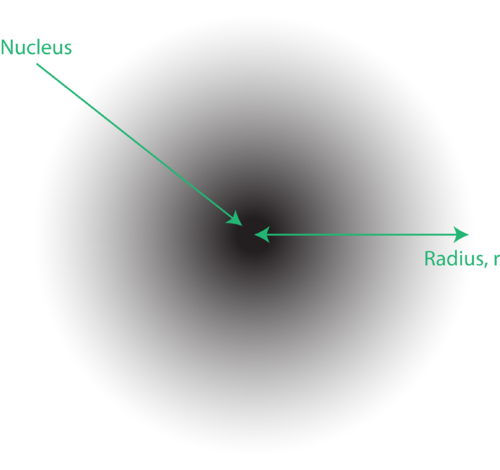## Quantum Mechanical Atomic Model

### Newsflash

The newsflash interrupts your favorite TV program. “There has been a hold-up at the First National Bank. The suspect fled in a car and is believed to be somewhere in the downtown district. Everyone is asked to be on the alert.” The robber can be located only within a certain area—the police do not have an exact location, just a general idea as to the whereabouts of the thief.

In 1926, Austrian physicist Erwin Schrödinger (1887–1961) used the wave-particle duality of the electron to develop and solve a complex mathematical equation that accurately described the behavior of the electron in a hydrogen atom. The quantum mechanical model of the atom comes from the solution to Schrödinger’s equation. Quantization of electron energies is a requirement in order to solve the equation. This is unlike the Bohr model, in which quantization was simply assumed with no mathematical basis.

Recall that in the Bohr model, the exact path of the electron was restricted to very well-defined circular orbits around the nucleus. The quantum mechanical model is a radical departure from that. Solutions to the Schrödinger wave equation, called wave functions , give only the probability of finding an electron at a given point around the nucleus. Electrons do not travel around the nucleus in simple circular orbits.Figure 1. An electron cloud: the darker region nearer the nucleus indicates a high probability of finding the electron, while the lighter region further from the nucleus indicates a lower probability of finding the electron.

The location of the electrons in the quantum mechanical model of the atom is often referred to as an electron cloud. The electron cloud can be thought of in the following way: Imagine placing a square piece of paper on the floor with a dot in the circle representing the nucleus. Now take a marker and drop it onto the paper repeatedly, making small marks at each point the marker hits. If you drop the marker many, many times, the overall pattern of dots will be roughly circular. If you aim toward the center reasonably well, there will be more dots near the nucleus and progressively fewer dots as you move away from it. Each dot represents a location where the electron could be at any given moment. Because of the uncertainty principle, there is no way to know exactly where the electron is. An electron cloud has variable densities: a high density where the electron is most likely to be and a low density where the electron is least likely to be (Figure 1).

In order to specifically define the shape of the cloud, it is customary to refer to the region of space within which there is a 90% probability of finding the electron. This is called an orbital , the three-dimensional region of space that indicates where there is a high probability of finding an electron.

### Summary

• The Schrödinger wave equation replaced the Bohr ideas about electron location with an uncertainty factor.
• The location of the electron can only be given as a probability that the electron is somewhere in a certain area.

### Practice

http://science.howstuffworks.com/atom8.htm

1. What was one problem with the Bohr model of the atom?
2. What did Heisenberg show about electrons?
3. What did Schrödinger derive?

### Review

1. What does the quantum mechanical view of the atom require?
2. What is a wave function?
3. What does a high density electron cloud suggest?

## Glossary

• electron cloud: The location of the electrons in the quantum mechanical model of the atom.
• orbital: The three-dimensional region of space that indicates where there is a high probability of finding an electron.
• quantum mechanical model: A model of the atom that derives from the Schrödinger wave equation and deals with probabilities.
• wave function: Give only the probability of finding an electron at a given point around the nucleus.